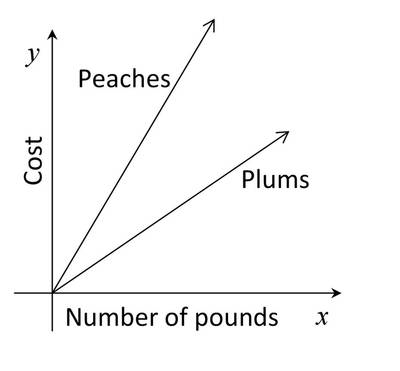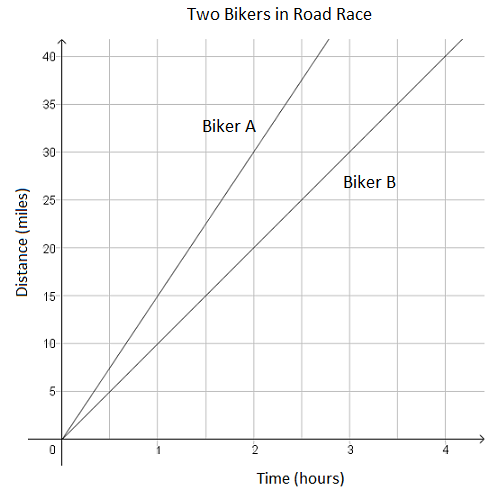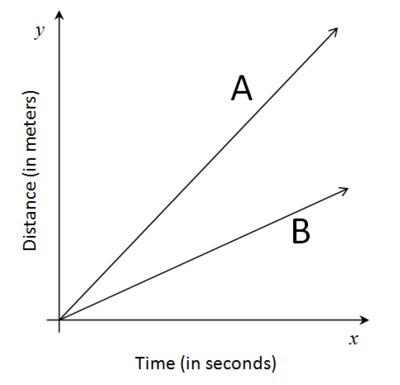# Linear Relationships

## Objective

Compare proportional relationships represented as graphs.

## Common Core Standards

### Core Standards

?

• 8.EE.B.5 — Graph proportional relationships, interpreting the unit rate as the slope of the graph. Compare two different proportional relationships represented in different ways. For example, compare a distance-time graph to a distance-time equation to determine which of two moving objects has greater speed.

?

• 7.RP.A.2

## Criteria for Success

?

1. In a graph with two proportional lines shown, determine which line has the greater slope or rate of change.
2. Understand that in proportional relationships, a graph with a steeper slope has a greater or higher rate of change.
3. Draw the graphs of two proportional situations in the same coordinate plane and use them to compare.

## Tips for Teachers

?

• The following materials are helpful for this lesson: graph paper.
• Lessons 3 and 4 address comparing proportional relationships. In the previous Unit 4, eighth-grade students compared functions across multiple representations by looking at the rates of change and the initial values. In this lesson, students look specifically at proportional relationships and bring in their new understanding of slope as the rate of change to understand how the relationships compare with one another. They focus on comparing multiple graphs, especially when presented in the same coordinate plane.

#### Fishtank Plus

• Problem Set
• Student Handout Editor
• Vocabulary Package

## Anchor Problems

?

### Problem 1

The graphs below show the cost $y$ of buying ${{x }}$ pounds of fruit. One graph shows the cost of buying ${{x }}$ pounds of peaches, and the other shows the cost of buying $x$ pounds of plums.1. Which kind of fruit costs more per pound? Explain.
2. Bananas cost less per pound than peaches or plums. Draw a line alongside the other graphs that might represent the cost, $y$, of buying $x$ pounds of bananas.

#### References

Illustrative Mathematics Peaches and Plums

Peaches and Plums, accessed on Feb. 23, 2018, 11:12 p.m., is licensed by Illustrative Mathematics under either the CC BY 4.0 or CC BY-NC-SA 4.0. For further information, contact Illustrative Mathematics.

### Problem 2

Kell works at an after-school program at an elementary school. The table below shows how much money he earned every day last week.

 Time worked (hours) 1.5 2.5 4 Money earned (dollars) 12.6 21 33.6

Mariko has a job mowing lawns that pays \$7 per hour.

Draw a graph for each boy’s situation, where $y$ represents the amount of money each boy earns for working $x$ hours. Your graph should have 2 lines drawn in it. Then answer the questions that follow.

1. Who makes more money for working 10 hours?
2. How can you see who makes more per hour by just looking at the graphs? Explain.
3. Use your graphs to determine who makes more for 2 hours of work and approximately how much more.

#### References

Illustrative Mathematics Who Has the Best Job?

Who Has the Best Job?, accessed on Feb. 23, 2018, 11:14 p.m., is licensed by Illustrative Mathematics under either the CC BY 4.0 or CC BY-NC-SA 4.0. For further information, contact Illustrative Mathematics.

Modified by The Match Foundation, Inc.

### Problem 3

The graph below shows the relationship between the distance biked and the time spent biking for two different bikers in a road race.Which of the following accurately compares the speed of the two bikers?

1. Biker A is going faster than Biker B by 5 miles per hour.
2. Biker A is going slower than Biker B by 5 miles per hour.
3. Biker A is going faster than Biker B by 10 miles per hour.
4. Biker A is going slower than Biker B by 10 miles per hour.

## Problem Set

?The following resources include problems and activities aligned to the objective of the lesson that can be used to create your own problem set.

• Include problems where students are given information about the rates of change in two or more situations and sketch the relative positions of each one on the same coordinate plane.

?

The graphs below show the distance two cars have traveled along the freeway over a period of several seconds. Car A is traveling 30 meters per second.Which equation from those shown below is the best choice for describing the distance traveled by car B after $x$ seconds? Explain.

1.   $y=85x$
2.   $y=60x$
3.   $y=30x$
4.   $y=15x$

#### References

Illustrative Mathematics Comparing Speeds in Graphs and Equations

Comparing Speeds in Graphs and Equations, accessed on Feb. 23, 2018, 11:19 p.m., is licensed by Illustrative Mathematics under either the CC BY 4.0 or CC BY-NC-SA 4.0. For further information, contact Illustrative Mathematics.

?